## Books : reviews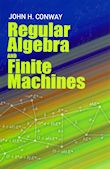### John Horton Conway.Regular Algebra and Finite Machines. Dover. 1971

Topics cover Moore’s theory of experiments, Kleene’s theory of regular events and expressions, Kleene algebras, the differential calculus of events, factors and the factor matrix, and the theory of operators. Additional subjects include event classes and operator classes, some regulator algebras, context-free languages, communicative regular algebra, axiomatic questions, the strength of classical axioms, and logical problems.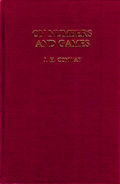### John Horton Conway.On Numbers and Games. Academic Press. 1976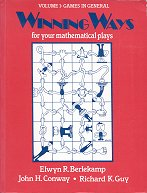### Elwyn R. Berlekamp, John Horton Conway, Richard K. Guy.Winning Ways for Your Mathematical Plays Volume 1: games in general. 1982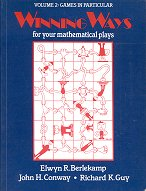### Elwyn R. Berlekamp, John Horton Conway, Richard K. Guy.Winning Ways for Your Mathematical Plays Volume 2: games in particular. 1982

Chapter 25 is devoted to the Game of Life.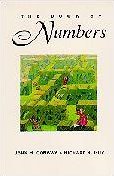### John Horton Conway, Richard K. Guy.The Book of Numbers. Copernicus / Springer. 1996

rating : 2.5 : great stuff
review : 22 June 1997

Journey through the world of numbers With two of the field’s most entertaining experts as your guides.

The Book of Numbers invites you to

• fraternize with famous families of numbers
• appreciate the primacy of primes
• fathom the fractions
• imagine imaginary numbers, and
• investigate the infinite ones.

This is a book unlike any other. Ranging from a fascinating survey of number names, words and symbols to an explanation of the new phenomenon of surreal numbers (“an extremely large yet infinitely small subclass of the most recent development of number theory”), The Book of Numbers is a fun and fascinating tour of numerical topics and concepts. It will have you contemplating ideas you never thought were understandable—or even possible.

This wonderful book is crammed with fascinating facts and properties about every kind of number. Many of the properties are given a geometric interpretation, so there are plenty of marvelous diagrams. Even if you’ve come across the material before, this geometric and diagrammatic approach may give you a new perspective on things --- for example, I was particularly taken with the ’telegraph pole’ representation of infinite ordinals in the last chapter. Many more weighty presentations tend to be short on diagrams. I think this is a pity: although ’proof by diagram’ is rarely sound, and nearly always needs to be backed up by a symbolic proof, diagrams themselves can do much to help prime the intuition.

The sign of a good book: I was fascinated by what was there, and was left wanting more. Here’s a brief overview of the contents, to give you a flavour of the breadth covered.

1. The Romance of Numbers. Names of numbers, and how number names influence the rest of language. Written number systems: Babylonian cuneiform, Greek, Roman, Egyptian, Hindu-Arabic. Other bases.
1. Doing Arithmetic and Algebra by Geometry. Square and triangular numbers, and other 2D shapes. Cube and tetrahedral numbers, and other 3D shapes (rhombic dodecahedral numbers, anyone?) 4D, and higher, shaped numbers. Wonderful geometric proofs of various equalities.

Tacked on the end comes a short section very large (finite) numbers. Fascinating, but I would have like a whole chapter on its own!
1. What Comes Next? Factorials, Pascal’s Triangle, binomial coefficients. Sequences, differencing, Jackson’s Difference fans.

2. Famous Families of Numbers. Bell numbers, Stirling numbers, Ramanujan’s numbers, Catalan numbers, Faulhaber’s formula, Bernoulli numbers, Euler numbers. Fibonacci numbers, why sunflower heads and pineapple rinds grow in a Fibonacci sequence.

3. The Primacy of Primes. Prime numbers, sieve of Eratosthenes. Arithmetic modulo p, unique factorisation, Mersenne numbers, perfect numbers, Fermat’s numbers and test for primes. Distribution of primes.

4. Further Fruitfulness of Fractions. Farey fractions, Ford circles, Euler’s totient numbers, decimal expansions, shuffling, Long primes, pythogorean fractions. Continued fractions.

5. Algebraic Numbers. Irrationality of root-2. Continued fractions of irrationals. Lagrange numbers, Markov numbers, Freiman’s number. Ruler and compass constructions, construction of regular 17-gon. Calbi’s triangle, Graham’s hexagon.

6. Imaginary Numbers. Complex plane, adding and multiplying. Gaussian integers and primes. The rational triangle. Hypercomplex numbers: Hamilton’s quaternions, Cayley numbers.

7. Transcendental numbers. Pi, Liouville’s number, Gregory’s numbers, Størmer’s numbers. Logarithms, e, harmonic numbers, Euler’s number, Stirling’s formula for n!

8. Infinite and infinitesimal numbers. Cantor’s ordinal numbers, cardinal numbers. Surreal numbers.